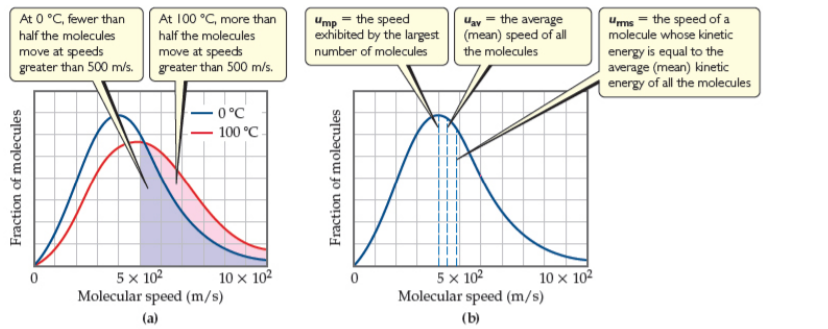# Problem: Distribution of molecular speeds for nitrogen gas. (a) The effect of temperature on molecular speed. The relative area under the curve for a range of speeds gives the relative fraction of molecules that have those speeds. (b) Position of most probable (ump), average (uav ), and root-mean-square (urms) speeds of gas molecules. The data shown here are for nitrogen gas at 0 °C. Estimate the fraction of molecules at 100 °C with speeds less than 300 m/s.a) about a quarter b) about a third c) about a half d) about one-sixth

###### FREE Expert Solution

Using the plot, we will estimate the fraction of molecules moving at speeds less than 300 m/s at 100°C (red line).

• x-axis  = speed in m/s
• y-axis =  fraction of molecules
100% (219 ratings)###### Problem Details

Distribution of molecular speeds for nitrogen gas. (a) The effect of temperature on molecular speed. The relative area under the curve for a range of speeds gives the relative fraction of molecules that have those speeds. (b) Position of most probable (ump), average (uav ), and root-mean-square (urms) speeds of gas molecules. The data shown here are for nitrogen gas at 0 °C.Estimate the fraction of molecules at 100 °C with speeds less than 300 m/s.

a) about a quarter

b) about a third

c) about a half

d) about one-sixth

Frequently Asked Questions

What scientific concept do you need to know in order to solve this problem?

Our tutors have indicated that to solve this problem you will need to apply the Velocity Distributions concept. You can view video lessons to learn Velocity Distributions. Or if you need more Velocity Distributions practice, you can also practice Velocity Distributions practice problems.

What professor is this problem relevant for?

Based on our data, we think this problem is relevant for Professor Halasyamani's class at UH.